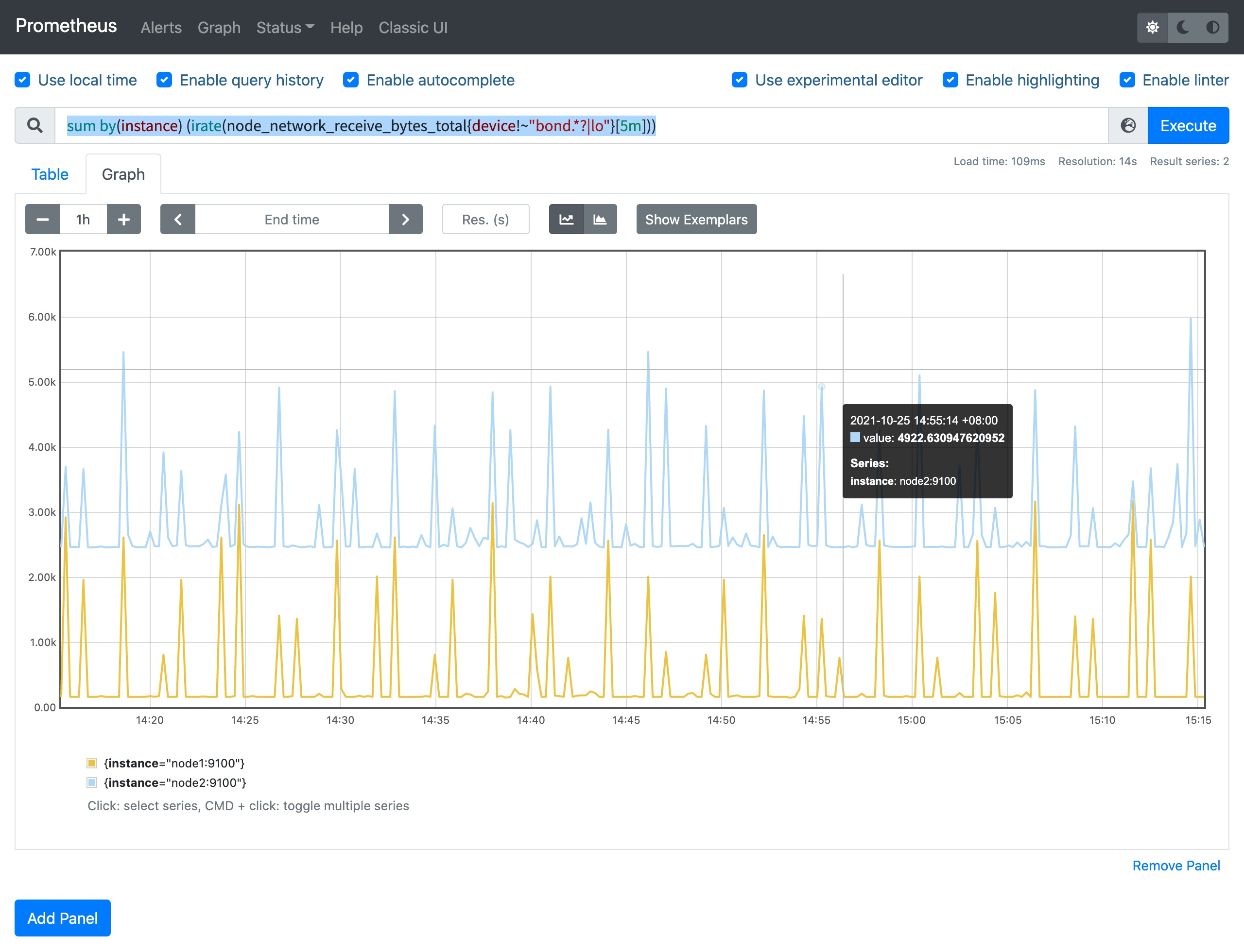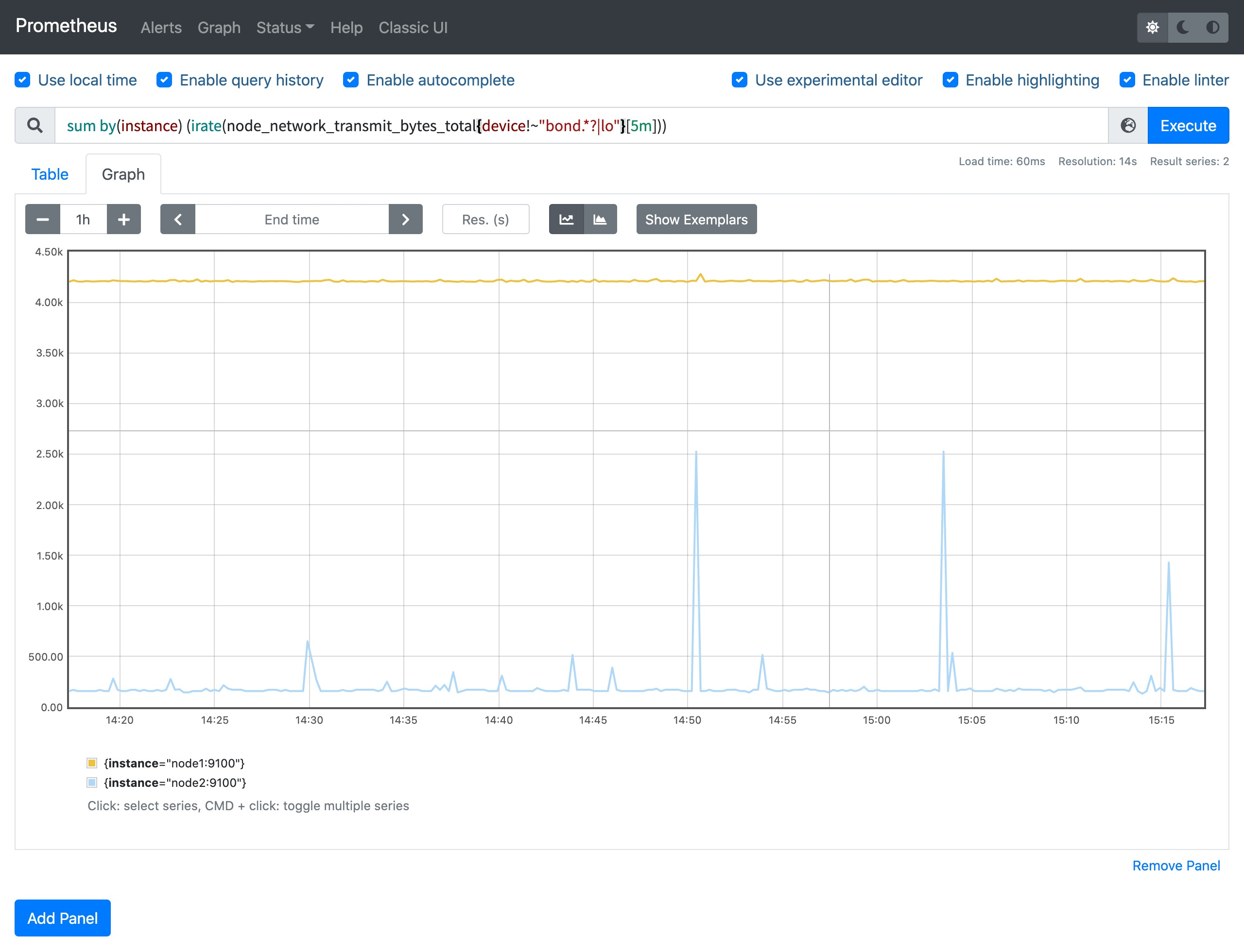# 常用监控指标¶

## CPU 监控¶

``````# HELP node_cpu_seconds_total Seconds the CPUs spent in each mode.
# TYPE node_cpu_seconds_total counter
node_cpu_seconds_total{cpu="0",mode="idle"} 13172.76
node_cpu_seconds_total{cpu="0",mode="iowait"} 0.25
node_cpu_seconds_total{cpu="0",mode="irq"} 0
node_cpu_seconds_total{cpu="0",mode="nice"} 0.01
node_cpu_seconds_total{cpu="0",mode="softirq"} 87.99
node_cpu_seconds_total{cpu="0",mode="steal"} 0
node_cpu_seconds_total{cpu="0",mode="system"} 309.38
node_cpu_seconds_total{cpu="0",mode="user"} 79.93
node_cpu_seconds_total{cpu="1",mode="idle"} 13168.98
node_cpu_seconds_total{cpu="1",mode="iowait"} 0.27
node_cpu_seconds_total{cpu="1",mode="irq"} 0
node_cpu_seconds_total{cpu="1",mode="nice"} 0
node_cpu_seconds_total{cpu="1",mode="softirq"} 74.1
node_cpu_seconds_total{cpu="1",mode="steal"} 0
node_cpu_seconds_total{cpu="1",mode="system"} 314.71
node_cpu_seconds_total{cpu="1",mode="user"} 78.83
node_cpu_seconds_total{cpu="2",mode="idle"} 13182.78
node_cpu_seconds_total{cpu="2",mode="iowait"} 0.69
node_cpu_seconds_total{cpu="2",mode="irq"} 0
node_cpu_seconds_total{cpu="2",mode="nice"} 0
node_cpu_seconds_total{cpu="2",mode="softirq"} 66.01
node_cpu_seconds_total{cpu="2",mode="steal"} 0
node_cpu_seconds_total{cpu="2",mode="system"} 309.09
node_cpu_seconds_total{cpu="2",mode="user"} 79.44
node_cpu_seconds_total{cpu="3",mode="idle"} 13185.13
node_cpu_seconds_total{cpu="3",mode="iowait"} 0.18
node_cpu_seconds_total{cpu="3",mode="irq"} 0
node_cpu_seconds_total{cpu="3",mode="nice"} 0
node_cpu_seconds_total{cpu="3",mode="softirq"} 64.49
node_cpu_seconds_total{cpu="3",mode="steal"} 0
node_cpu_seconds_total{cpu="3",mode="system"} 305.86
node_cpu_seconds_total{cpu="3",mode="user"} 78.17
``````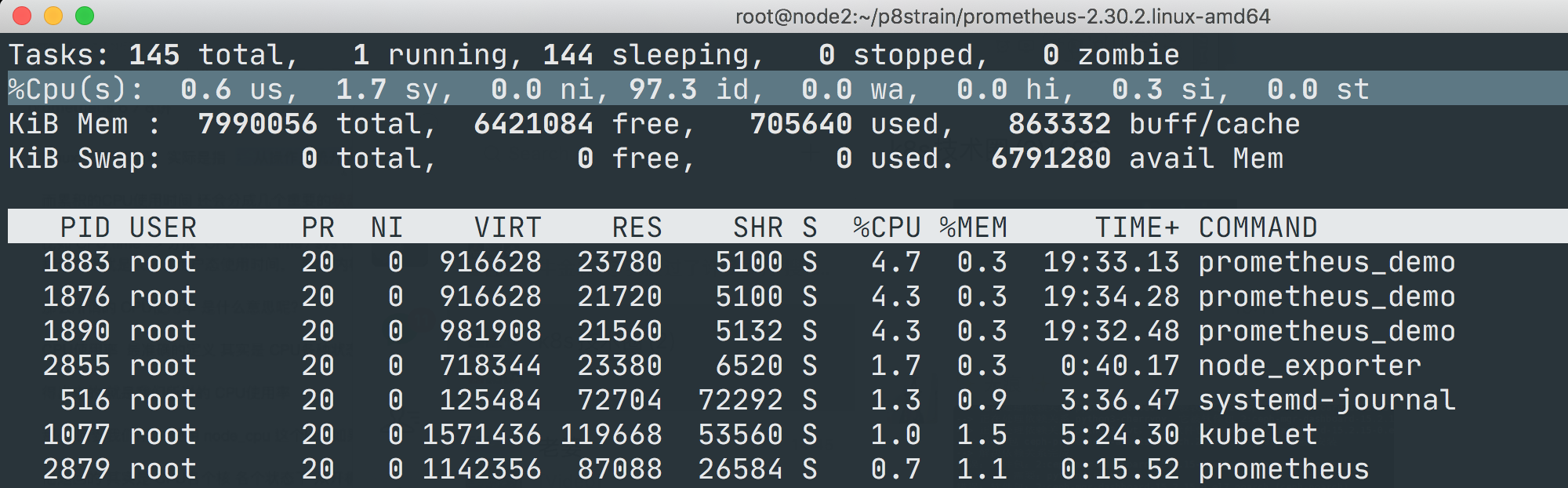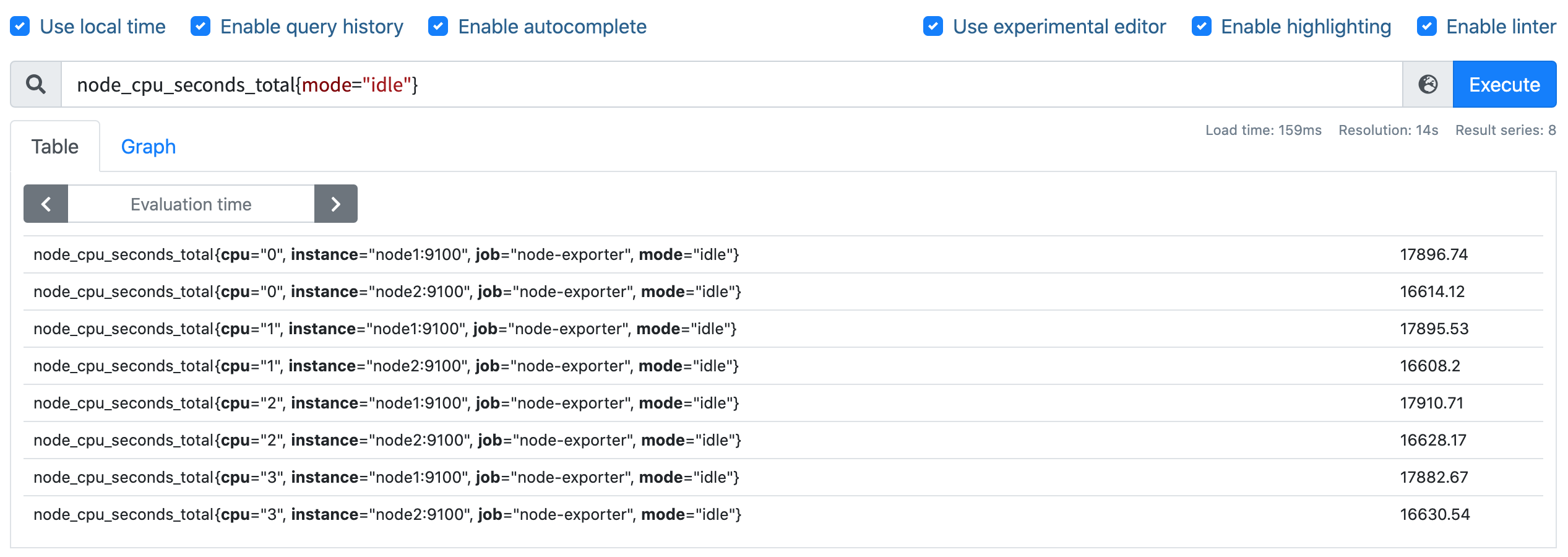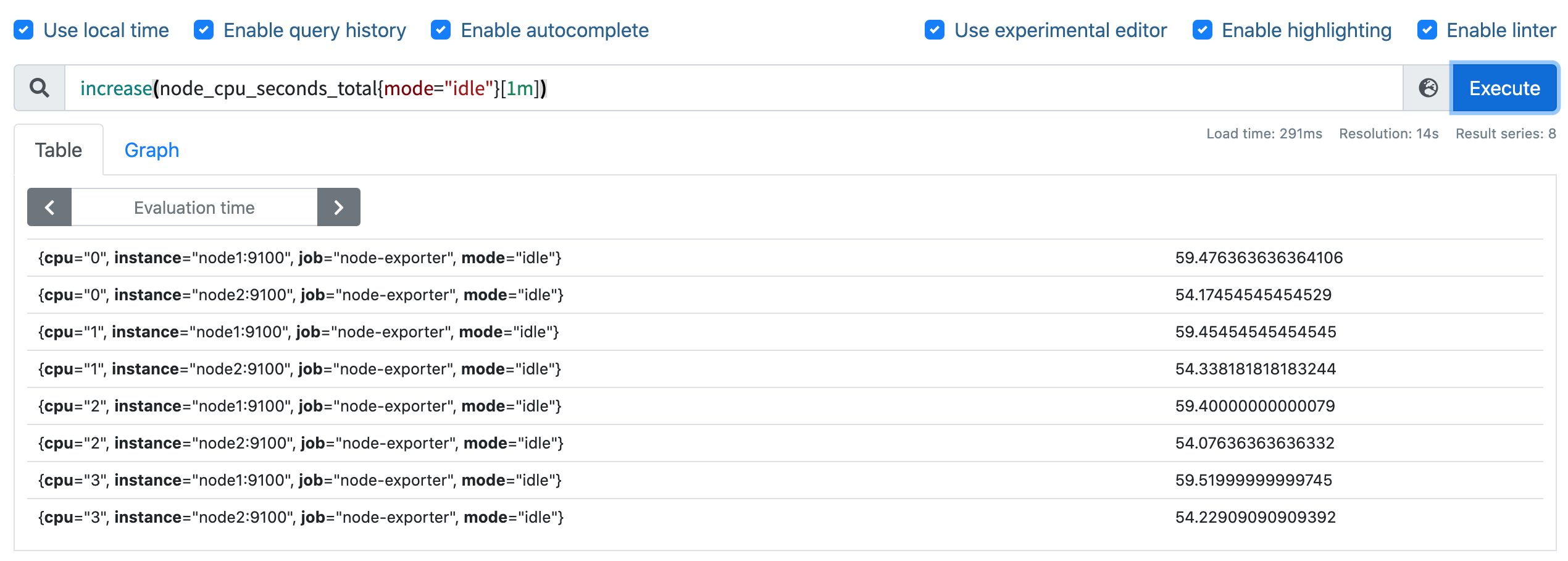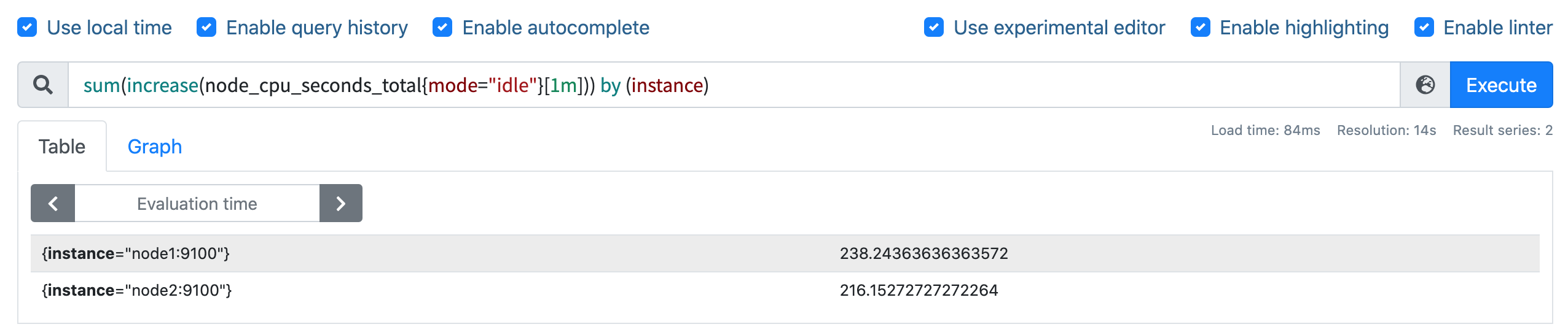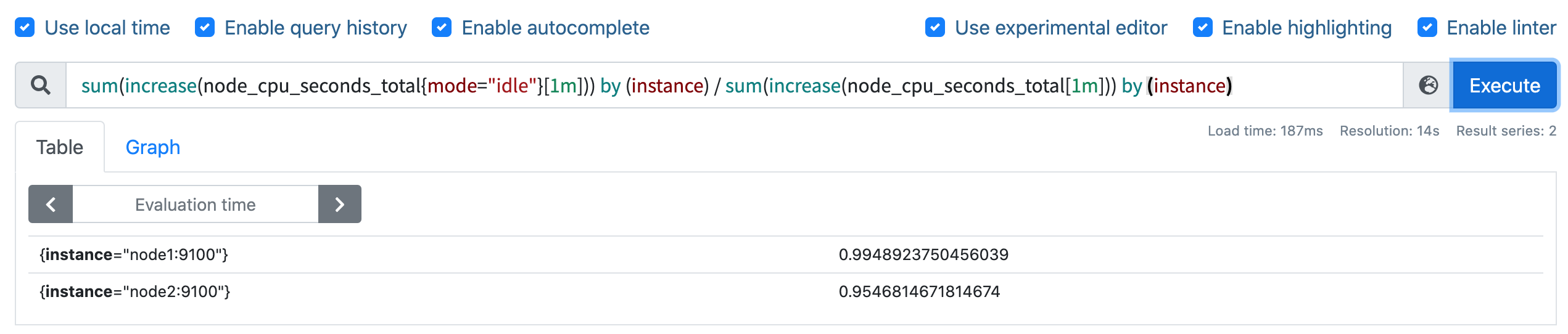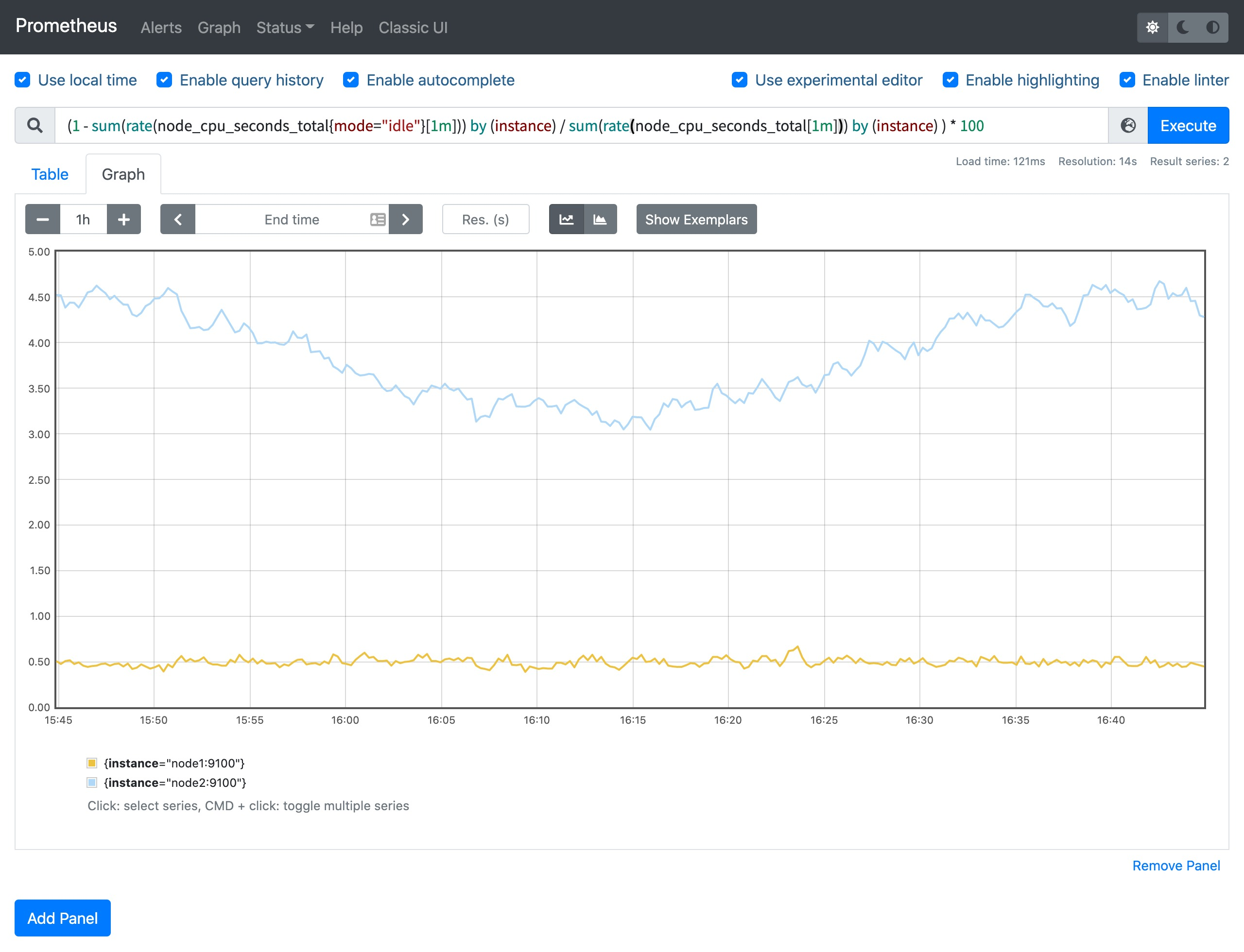## 内存监控¶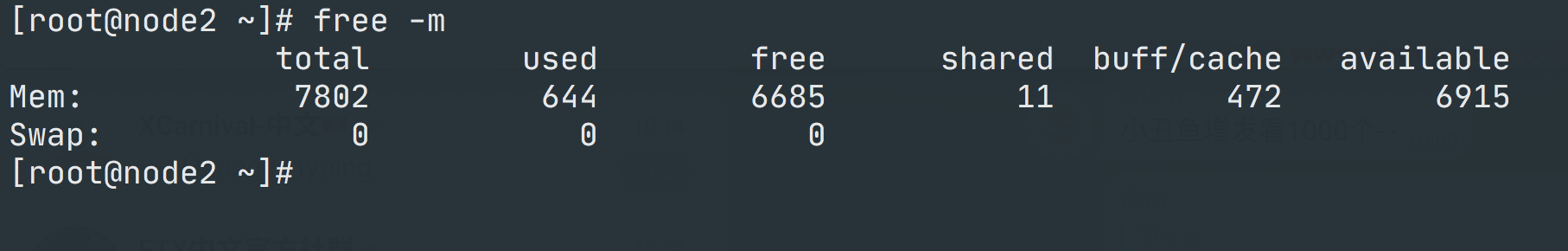`free` 命令的输出会显示系统内存的使用情况，包括物理内存、交换内存(swap)和内核缓冲区内存等，所以要对内存进行监控我们需要先了解这些概念，我们先了解下 `free` 命令的输出内容：

• `Mem 行`(第二行)是内存的使用情况
• `Swap 行`(第三行)是交换空间的使用情况
• `total` 列显示系统总的可用物理内存和交换空间大小
• `used` 列显示已经被使用的物理内存和交换空间
• `free` 列显示还有多少物理内存和交换空间可用使用
• `shared` 列显示被共享使用的物理内存大小
• `buff/cache` 列显示被 buffer 和 cache 使用的物理内存大小
• `available` 列显示还可以被应用程序使用的物理内存大小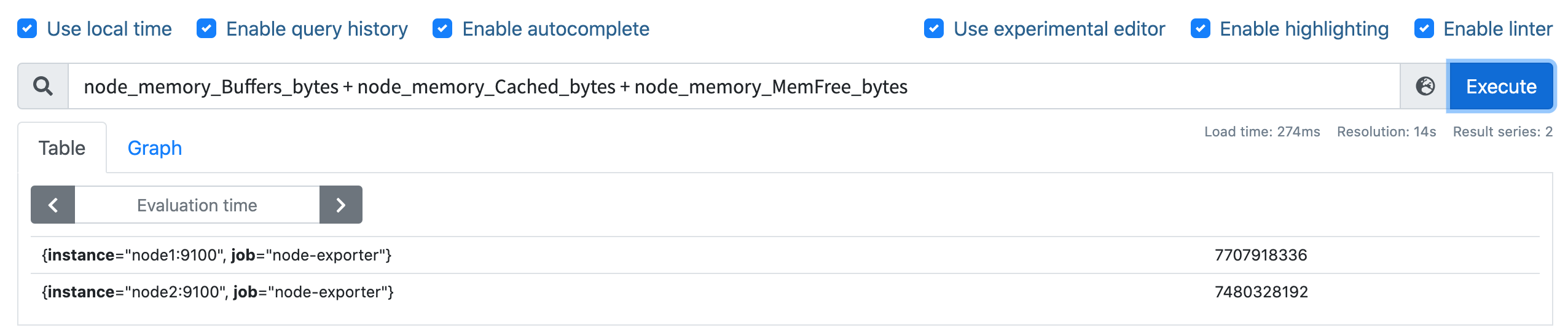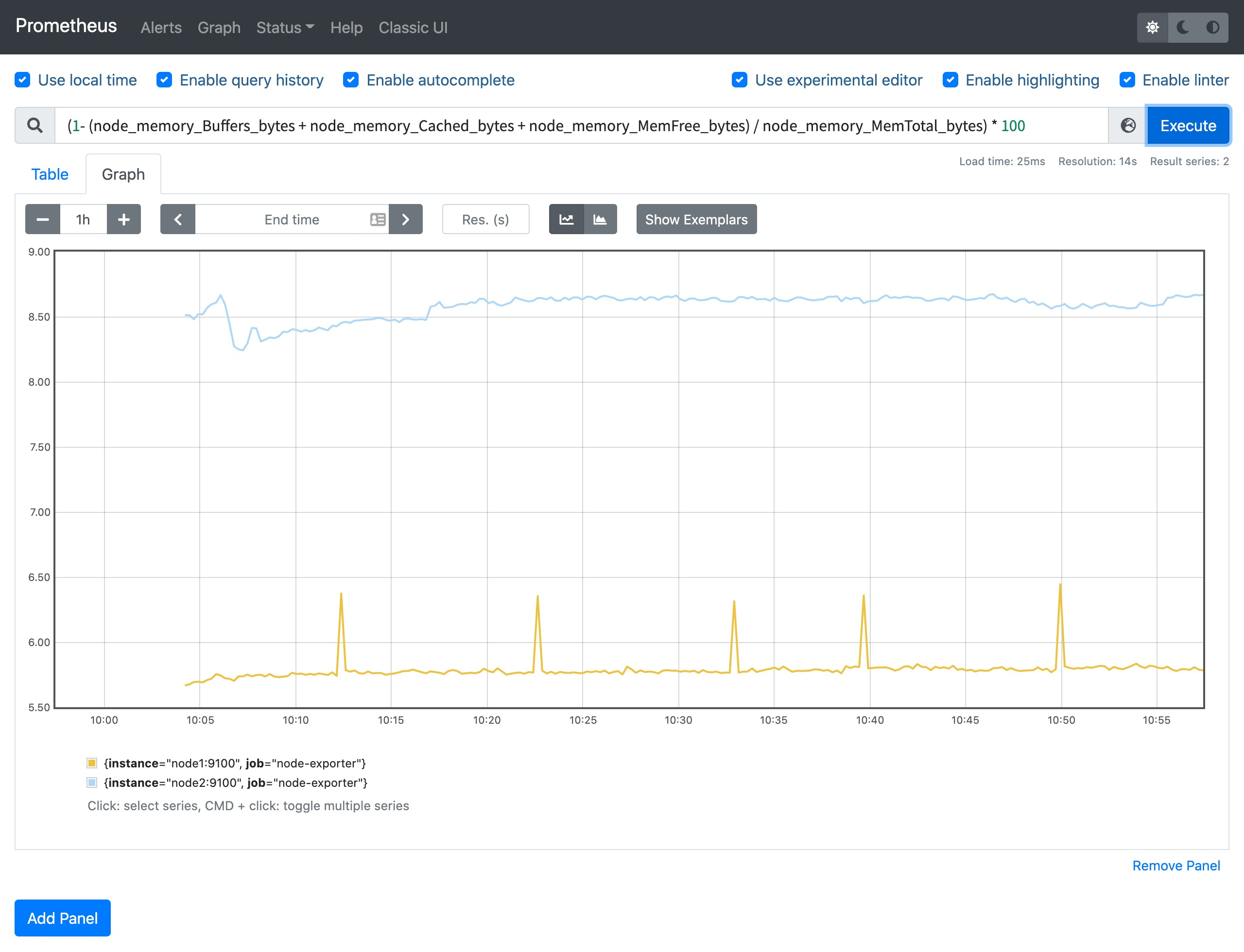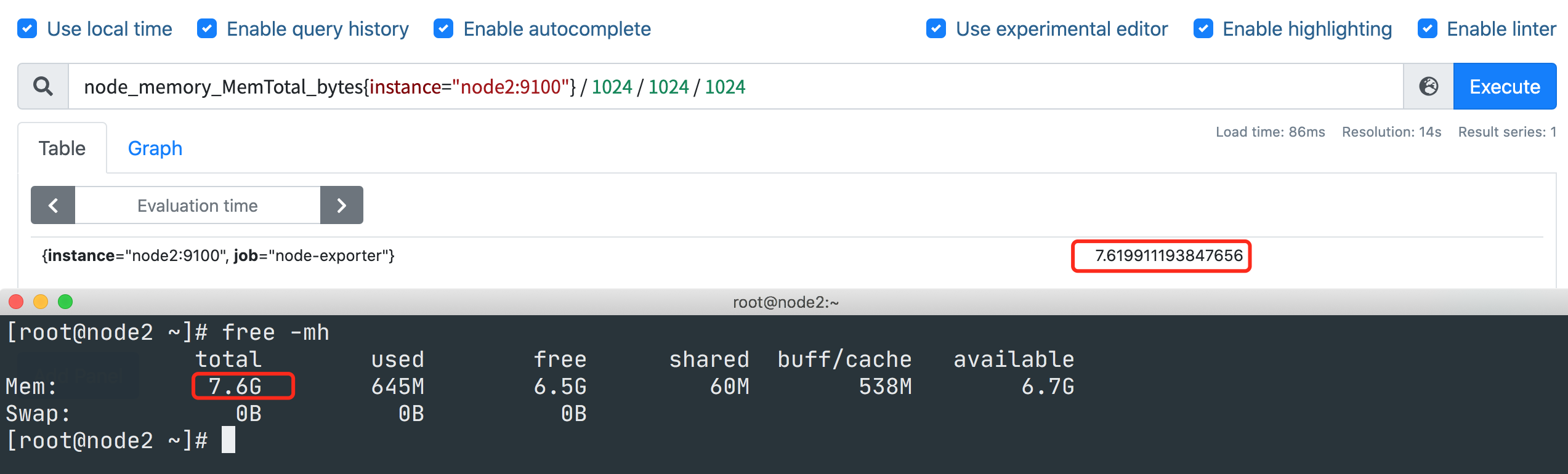## 磁盘监控¶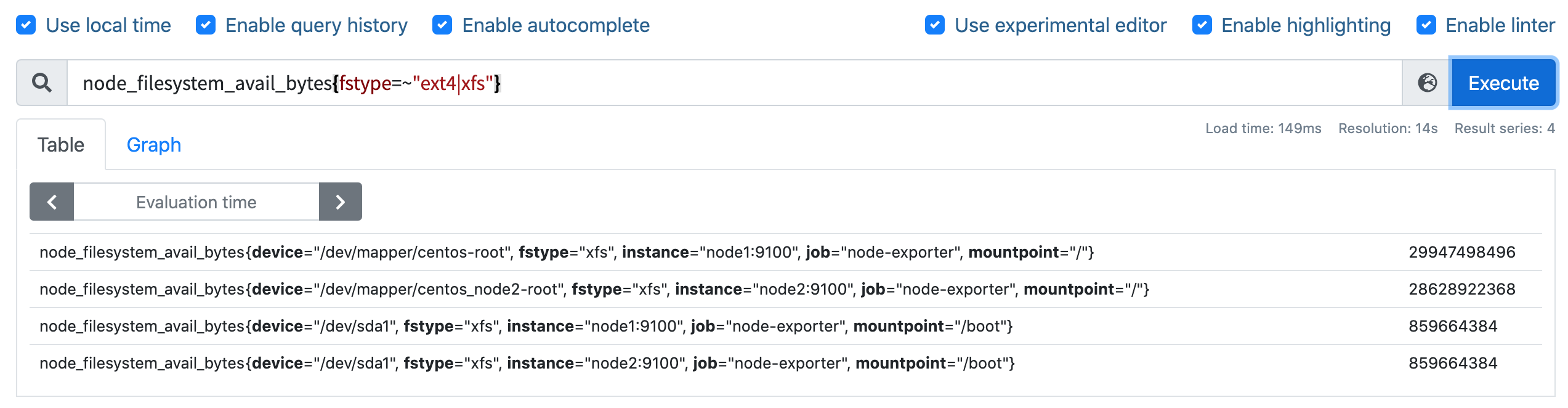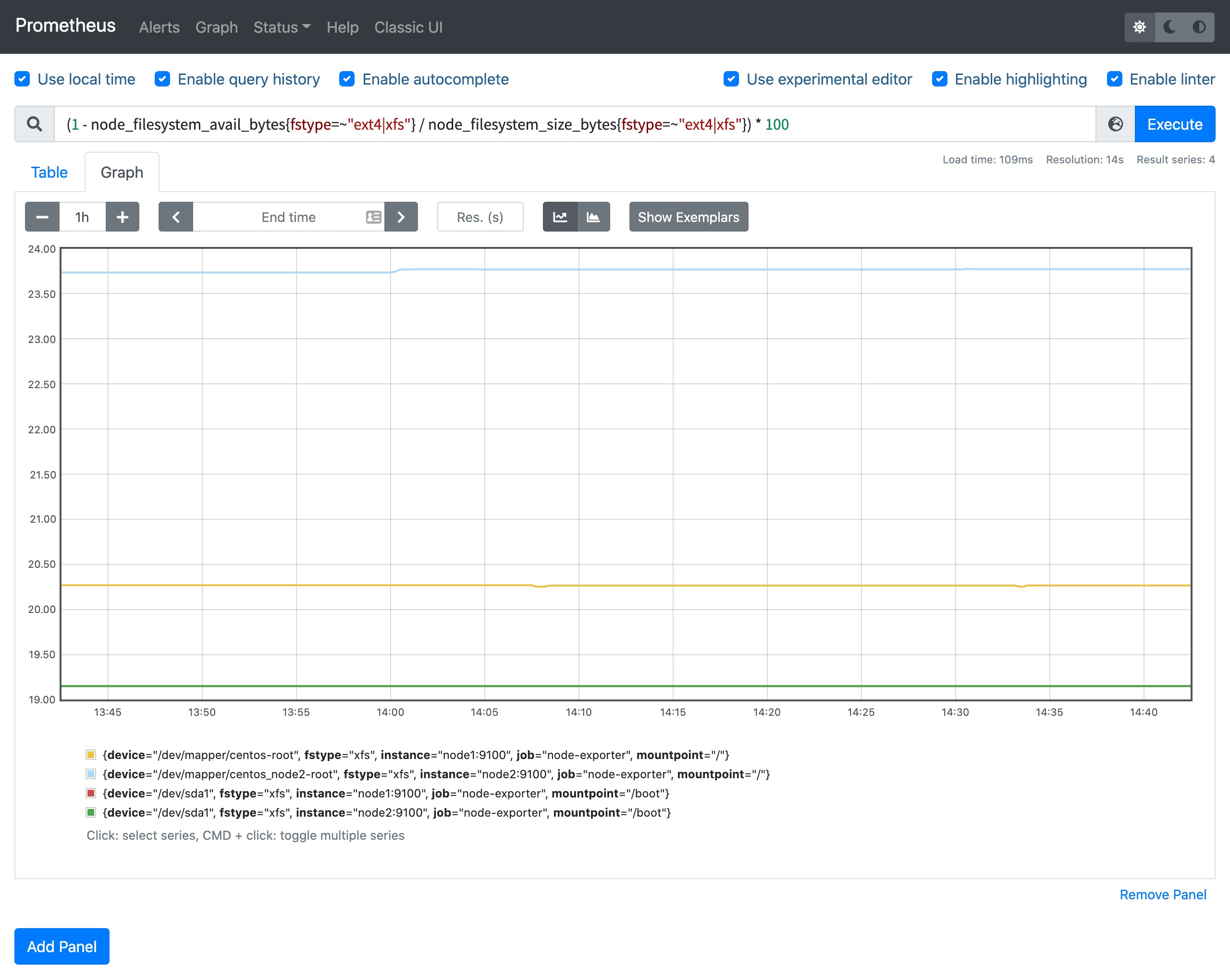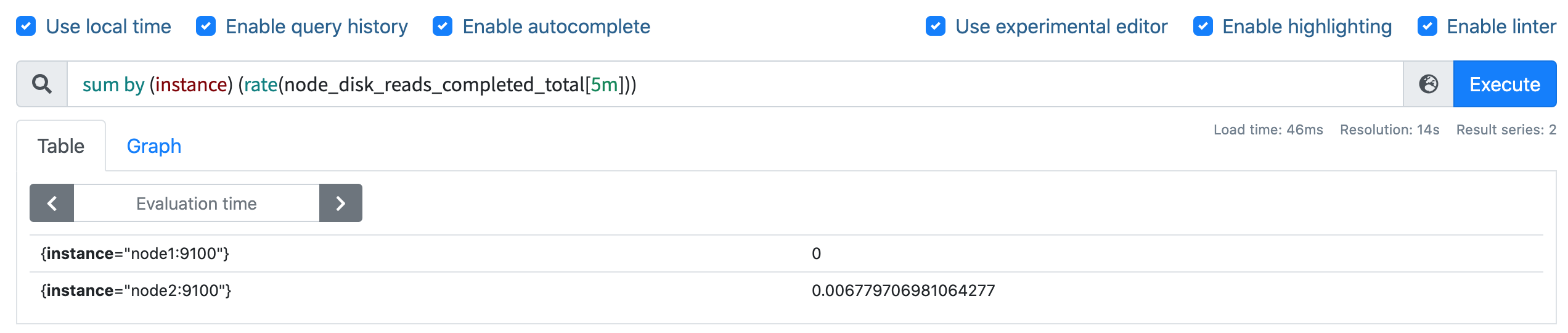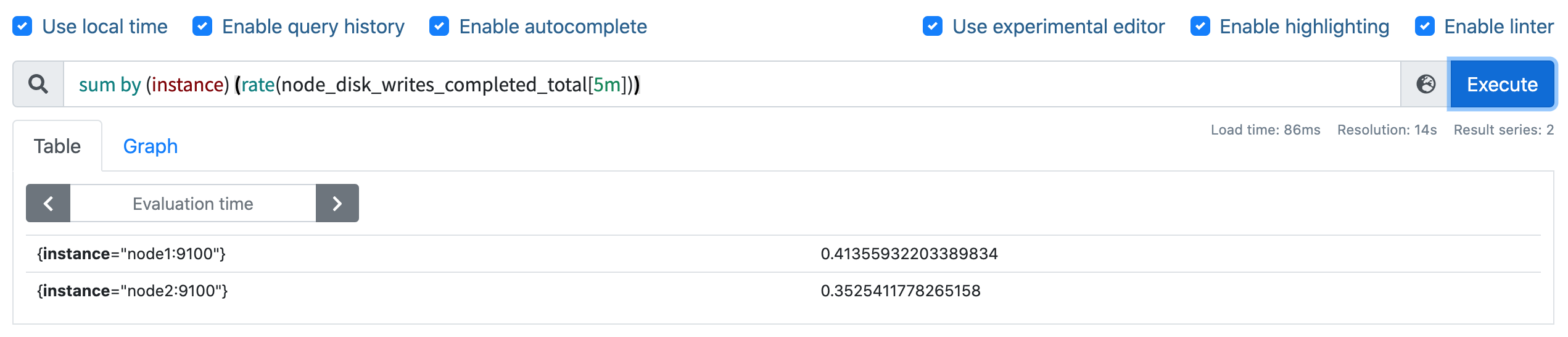## 网络 IO 监控¶